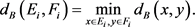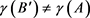# 基于正负类边界距离的多标签数据属性约简Attribute Reduction for Multi-Label Data Based on Boundary Distance between Positive and Negative Classes

DOI: 10.12677/CSA.2020.109163, PDF, HTML, XML, 下载: 176  浏览: 307

Abstract: In this paper, the multi-label classification problem is decomposed into a series of single-label bi-nary classification sub-problems, each of which corresponds to one label. The positive class of the sub-problem is defined as the sample with the label, and the negative class is defined as the sample without the label. Under the given attribute subset, the minimum distance between positive and negative class samples is calculated, that is, the minimum distance of classification boundary. The sum of the boundary distances for all sub-problems is defined as dependency function, which is used as the evaluation of the importance of the attribute subset. The monotonicity of the proposed dependence function with respect to the attribute subset is established, and the definition of the attribute reduction is given by maximizing the dependence function. Finally, an attribute reduction algorithm based on positive and negative class boundary distance is designed, and the experiments are carried out on actual multi-label data sets. The experimental results show that the proposed algorithm can establish a reasonable ranking of attribute importance and effectively remove redundant attributes.

1. 引言

2. 基础知识

${d}_{B}\left(x,y\right)=\underset{a\in B}{\sum }|a\left(x\right)-a\left(y\right)|.$

${d}_{B}\left(X,Y\right)=\underset{x\in X,y\in Y}{\mathrm{min}}{d}_{B}\left(x,y\right).$

${d}_{B}\left(x,Y\right)=\underset{y\in Y}{\mathrm{min}}{d}_{B}\left(x,y\right).$

3. 多标签数据属性约简

${E}_{i}=\left\{x\in U:{l}_{i}\left(x\right)=1\right\},i=1,\cdots ,q.$

${F}_{i}=\left\{x\in U:{l}_{i}\left(x\right)=\text{0}\right\},i=1,\cdots ,q.$$\gamma \left(B\right)=\underset{i=1}{\overset{q}{\sum }}{d}_{B}\left({E}_{i},{F}_{i}\right)$

${d}_{{B}_{\text{1}}}\left(x,y\right)=\underset{a\in {B}_{\text{1}}}{\sum }|a\left(x\right)-a\left(y\right)|\le \underset{a\in {B}_{2}}{\sum }|a\left(x\right)-a\left(y\right)|={d}_{{B}_{2}}\left(x,y\right)$

${d}_{{B}_{1}}\left({E}_{i},{F}_{i}\right)=\underset{x\in {E}_{i},y\in {F}_{i}}{\mathrm{min}}{d}_{{B}_{1}}\left(x,y\right)\le {d}_{{B}_{1}}\left(x,y\right)$

${d}_{{B}_{\text{2}}}\left({E}_{i},{F}_{i}\right)=\underset{x\in {E}_{i},y\in {F}_{i}}{\mathrm{min}}{d}_{{B}_{\text{2}}}\left(x,y\right)\le {d}_{{B}_{\text{2}}}\left(x,y\right)$

${d}_{{B}_{2}}\left({E}_{i},{F}_{i}\right)-{d}_{{B}_{\text{1}}}\left({E}_{i}-{F}_{i}\right)>0$

${d}_{{B}_{1}}\left({E}_{i},{F}_{i}\right)<{d}_{{B}_{2}}\left({E}_{i},{F}_{i}\right)$

$\gamma \left({B}_{1}\right)=\underset{i=1}{\overset{q}{\sum }}{d}_{{B}_{1}}\left({E}_{i},{F}_{i}\right)$

$\gamma \left({B}_{2}\right)=\underset{i=1}{\overset{q}{\sum }}{d}_{{B}_{2}}\left({E}_{i},{F}_{i}\right)$

$\underset{i=1}{\overset{q}{\sum }}{d}_{{B}_{2}}\left({E}_{i},{F}_{i}\right)-\underset{i=1}{\overset{q}{\sum }}{d}_{{B}_{1}}\left({E}_{i},{F}_{i}\right)\ge 0$

$\gamma \left({B}_{1}\right)\le \gamma \left( B 2 \right)$

1) $\gamma \left(B\right)=\gamma \left(A\right)$

2) 对于任意的 ${B}^{\prime }\subset B$1) $|\gamma \left(B\right)-\gamma \left(A\right)|<\epsilon$

2) 对于任意的 ${B}^{\prime }\subset B$$|\gamma \left({B}^{\prime }\right)-\gamma \left(A\right)|\ge \epsilon$

4. 实验Table 6. Average numbers of the selected attributes

F1 score相对于汉明损失是一个综合版本，F1 score衡量分类的准确性，并忽略错误分类的样本。它是精确率和召回率的调和平均值，该指标取值越大，算法的性能越好，通常最大值为1，最小值为0，当值为1时达到最优。从表9可知，除数据集Core15k外，五种算法在F1 score方面没有显著差异。对于Core15k数据集，NLD算法和MDMR算法的分类精度与原始数据差不多。

5. 总结

  Zhang, M.L. and Zhou, Z.H. (2014) A Review on Multi-Label Learning Algorithms. IEEE Transactions on Knowledge and Data Engineering, 26, 1819-1837. https://doi.org/10.1109/TKDE.2013.39  Xu, S.P., Yang, X.B., Yu, H.L., Yu, D.J., Yang, J.Y., Eric, C.C. and Tsang, J. (2016) Multi-Label Learning with Label-Specific Feature Reduction. Knowledge-Based Systems, 104, 52-61. https://doi.org/10.1016/j.knosys.2016.04.012  Zhu, P.F., Xu, Q., Hu, Q.H., Zhang, C.Q. and Zhao, H. (2018) Multi-Label Feature Selection with Missing Labels. Pattern Recognition, 74, 488-502. https://doi.org/10.1016/j.patcog.2017.09.036  Li, H., Li, D.Y., Zhai, Y.H., Wang, S.G. and Zhang, J. (2016) A Novel Attribute Reduction Approach for Multi-Label Data Based on Rough Set Theory. Information Sciences, 367-368, 827-847. https://doi.org/10.1016/j.ins.2016.07.008  Li, Y.W., Lin, Y.J., Liu, J.H., Weng, W., Shi, Z.K. and Wu, S.X. (2018) Feature Selection for Multi-Label Learning Based on Kernelized Fuzzy Rough Sets. Neurocomputing, 318, 271-286. https://doi.org/10.1016/j.neucom.2018.08.065  Liu, J.H., Lin, Y.J., Li, Y.W., Weng, W. and Wu, S.X. (2018) Online Multi-Label Streaming Feature Selection Based on Neighborhood Rough Set. Pattern Recognition, 84, 273-287. https://doi.org/10.1016/j.patcog.2018.07.021  Lin, Y.J., Hu, Q.H., Zhang, J. and Wu, X.D. (2016) Mul-ti-Label Feature Selection with Streaming Labels. Information Sciences, 372, 256-275. https://doi.org/10.1016/j.ins.2016.08.039  Fan, X.D., Zhao, W.D., Wang, C.Z. and Huang, Y. (2018) Attribute Re-duction Based on Max-Decision Neighborhood Rough Set Model. Knowledge-Based Systems, 151, 16-23. https://doi.org/10.1016/j.knosys.2018.03.015  Lin, Y.J., Li, Y.W., Wang, C.X. and Chen, J.K. (2018) Attribute Re-duction for Multi-Label Learning with Fuzzy Rough Set. Knowledge-Based Systems, 152, 51-61. https://doi.org/10.1016/j.knosys.2018.04.004  Wang, C.X., Lin, Y.J. and Liu, J.H. (2019) Feature Selection for Multi-Label Learning with Missing Labels. Applied Intelligence, 49, 3027-3042. https://doi.org/10.1007/s10489-019-01431-6  Liu, J.H., Li, Y.W., Weng, W., Zhang, J., Chen, B.H. and Wu, S.X. (2020) Feature Selection for Multi-Label Learning with Streaming Label. Neurocomputing, 387, 268-278. https://doi.org/10.1016/j.neucom.2020.01.005  段洁, 胡清华, 张灵均, 钱宇华, 李德玉. 基于邻域粗糙集的多标记分类特征选择算法[J]. 计算机研究与发展, 2015, 52(1): 56-65.  Lin, Y.J., Hu, Q.H., Liu, J.H. and Duan, J. (2015) Multi-Label Feature Selection Based on Max-Dependency and Min-Redundancy. Neurocomputing, 168, 92-103. https://doi.org/10.1016/j.neucom.2015.06.010  Fan, X.D., Chen, Q., Qiao, Z.J., Wang, C.Z. and Ten, M.Y. (2020) Attribute Reduction for Multi-Label Classification Based on Labels of Positive Region. Soft Computing, 1-11. https://doi.org/10.1007/s00500-020-04780-4  Zhang, M.L. and Zhou, Z.H. (2006) ML-KNN: A Lazy Learning Approach to Multi-Label Learning. Pattern Recognition, 40, 2038-2048. https://doi.org/10.1016/j.patcog.2006.12.019